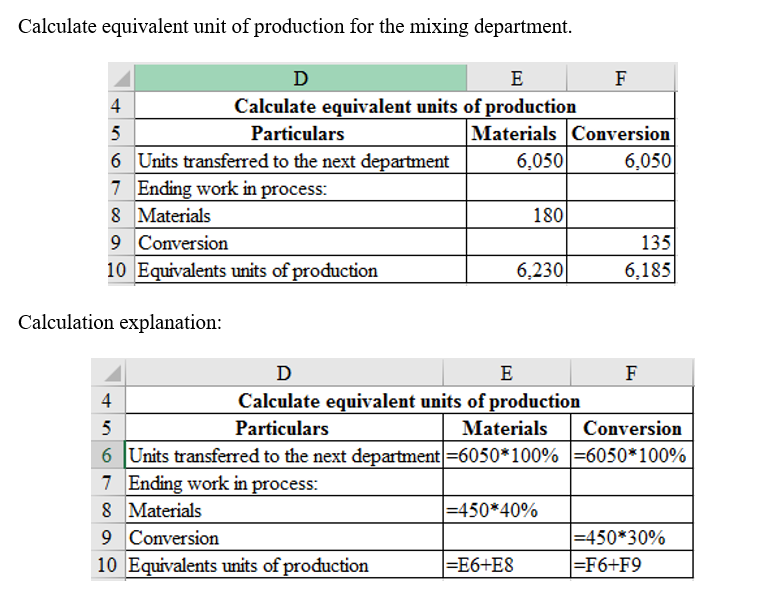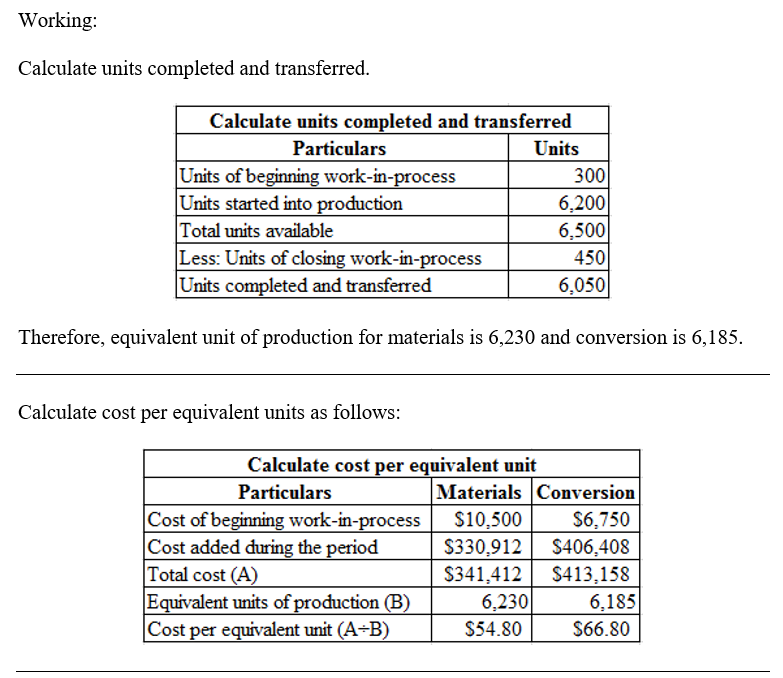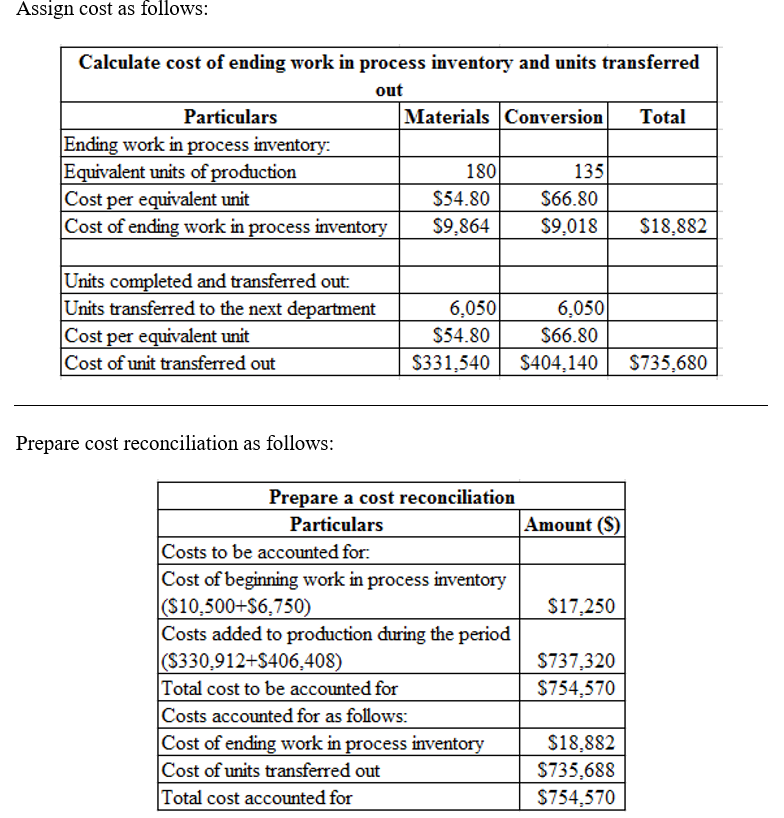# Question & Answer: Hayword, Inc. uses weighted-average costing and has two departments and has provided data related to its mixing department for the…..

Hayword, Inc. uses weighted-average costing and has two departments and has provided data related to its mixing department for the month of July. The Controller has asked you prepare a cost reconciliation report and provide the related computations. Use the information included in the Excel Simulation and the Excel functions described below to complete the task.

• Cell Reference: Allows you to refer to data from another cell in the worksheet. From the Excel Simulation below, if in a blank cell, “=B5” was entered, the formula would output the result from cell B5, or 300 in this example.
• Basic Math functions: Allows you to use the basic math symbols to perform mathematical functions. You can use the following keys: + (plus sign to add), – (minus sign to subtract), * (asterisk sign to multiply), and / (forward slash to divide). From the Excel Simulation below, if in a blank cell “=B9+B10” was entered, the formula would add the values from those cells and output the result, or 17,250 in this example. If using the other math symbols the result would output an appropriate answer for its function.
• SUM function: Allows you to refer to multiple cells and adds all the values. You can add individual cell references or ranges to utilize this function. From the Excel Simulation below, if in a blank cell “=SUM(B13,B14)” was entered, the formula would output the result of adding those two separate cells, or 737,320 in this example. Similarly, if in a blank cell “=SUM(B13:B14)” was entered, the formula would output the same result of adding those cells, except they are expressed as a range in the formula, and the result would be 737,320 in this example.​
•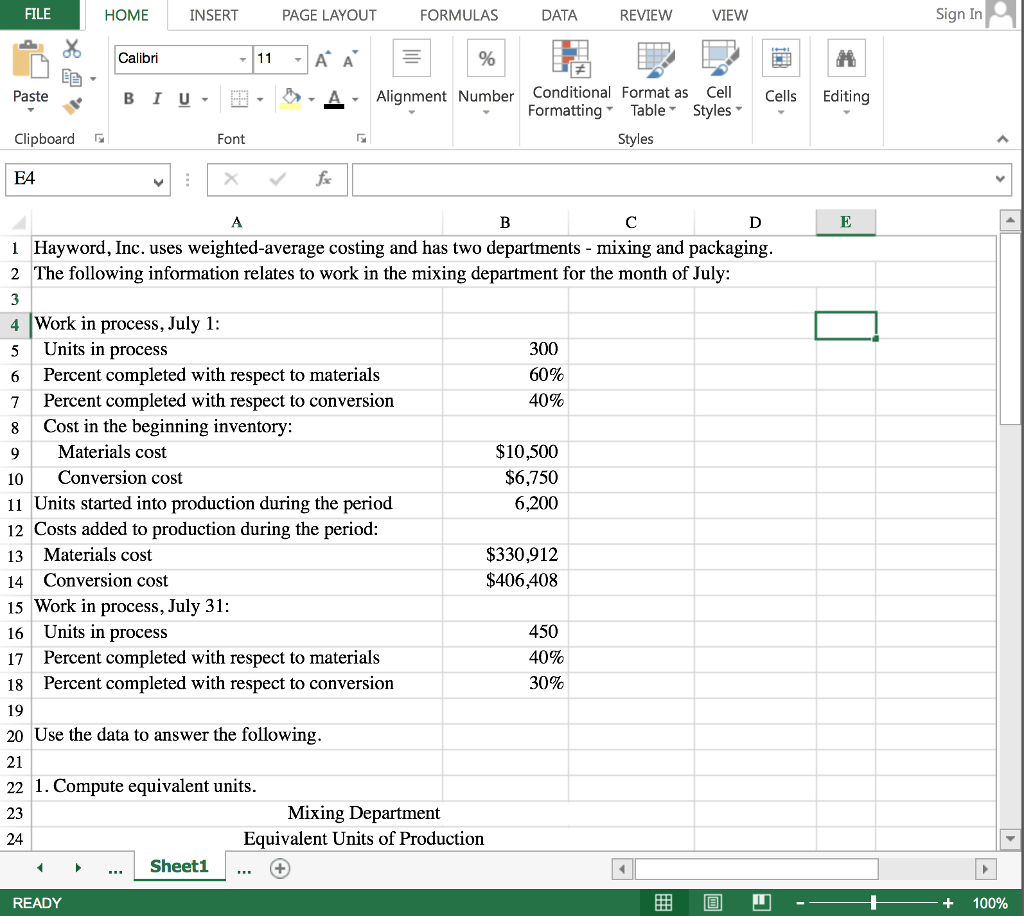•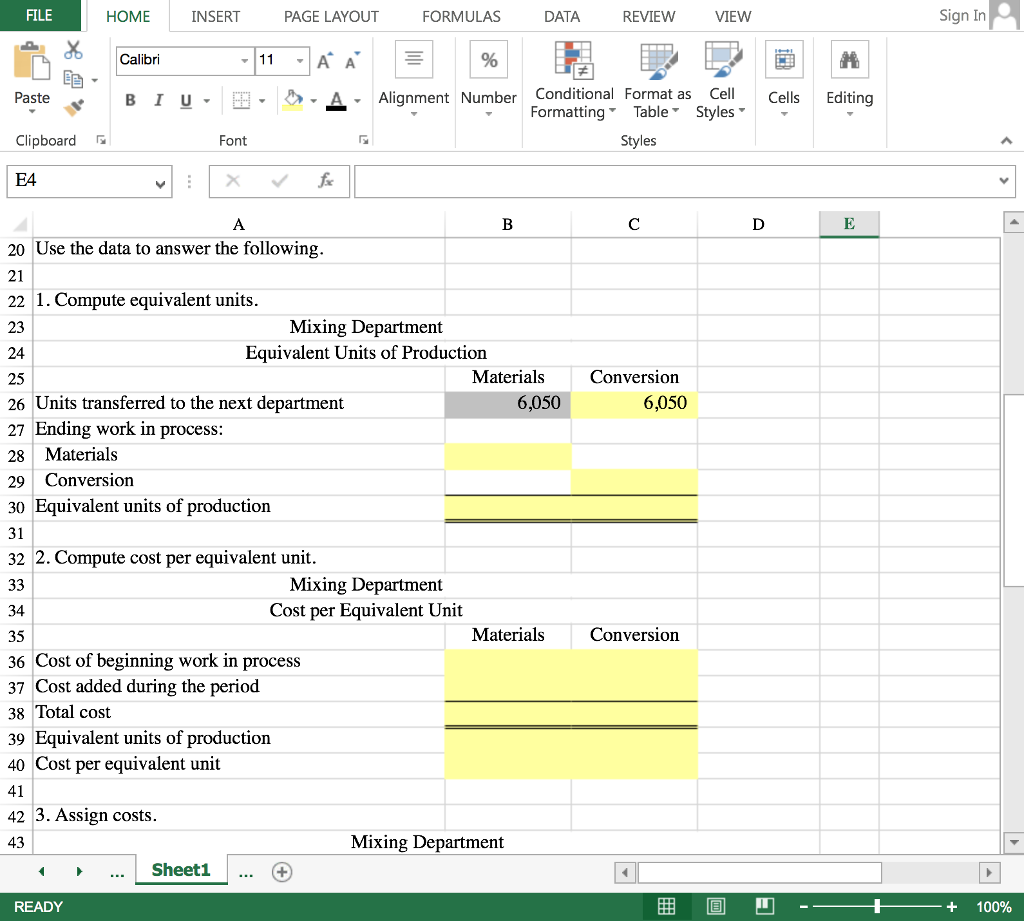•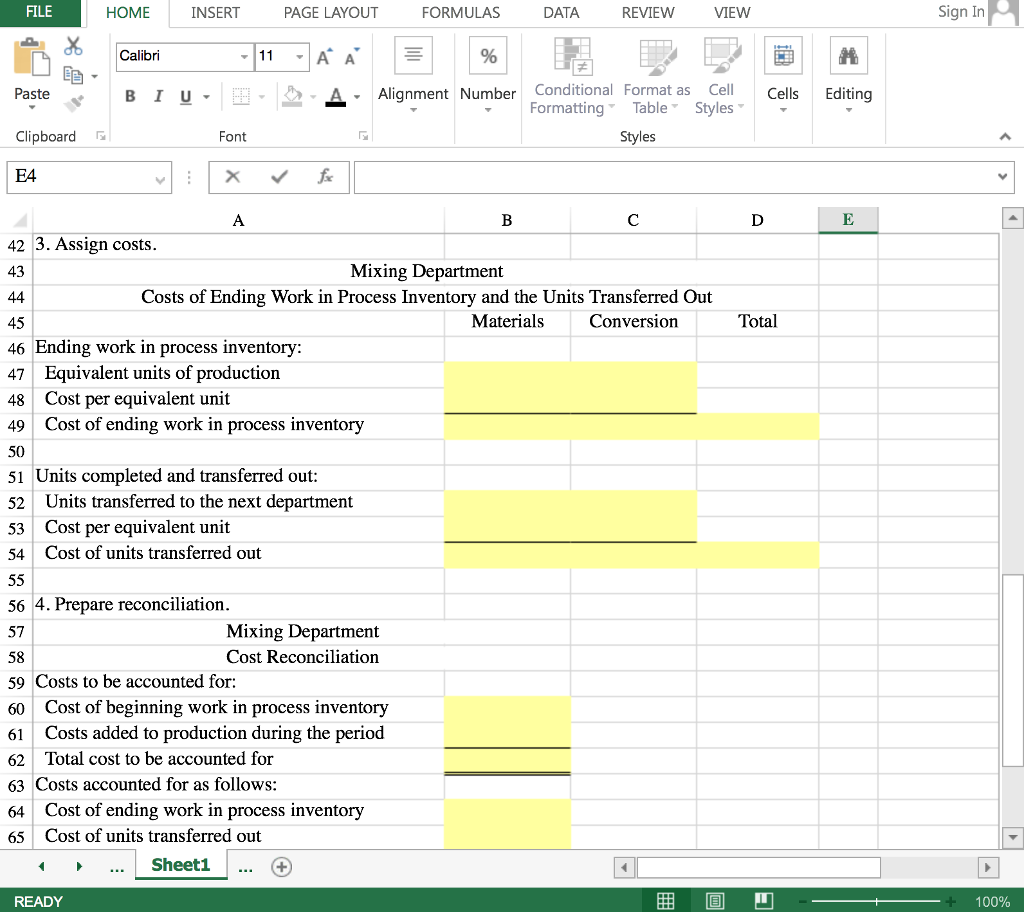•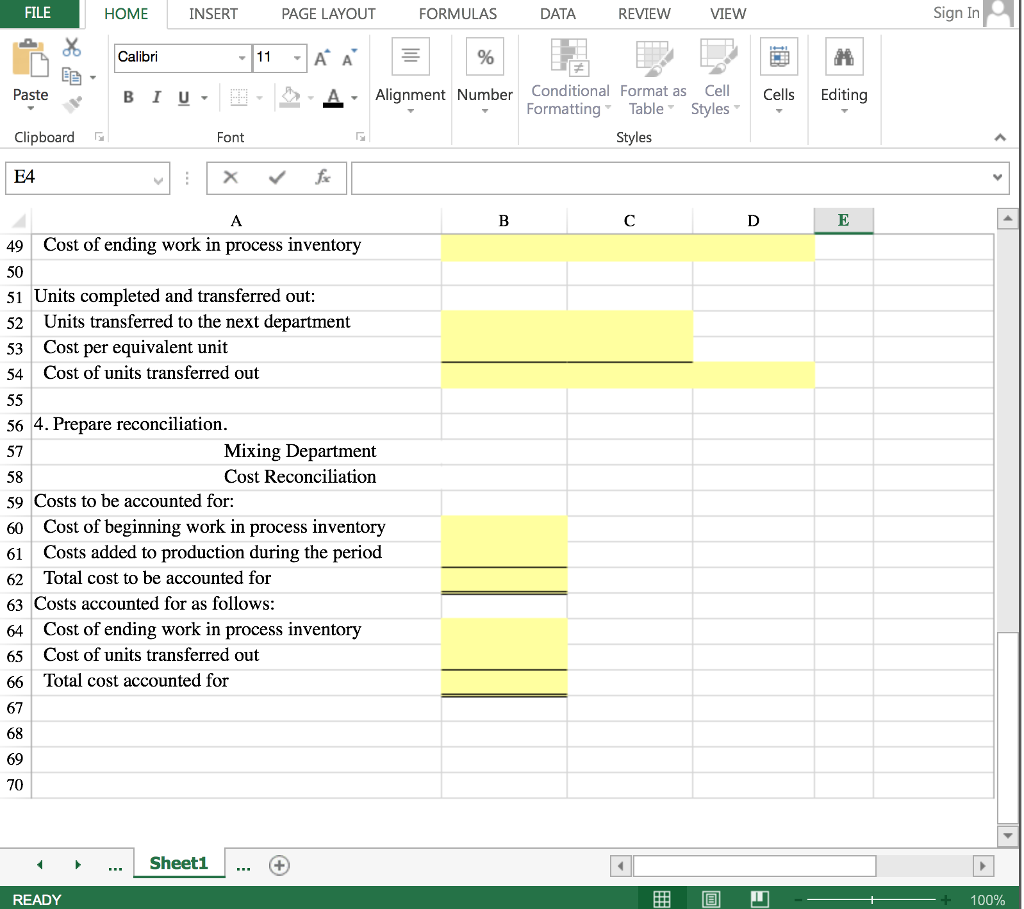FILE HOME INSERT PAGE LAYOUT FORMULAS DATA REVIEW VIEW Sign In Calibri Alignment Number Conditional Format as Cell Cells Editing Paste Clipboard E4 B l u. 田 ク Formatting TableStyles- Font es 1Hayword, Inc. uses weighted-average costing and has two departments – mixing and packaging 2 The following information relates to work in the mixing department for the month of July 4 |Work in process, July 1: 5 Units in process 6 Percent completed with respect to materials 7Percent completed with respect to conversion 8 Cost in the beginning inventory 9Materials cost 10 Conversion cost 11 Units started into production during the period 12 Costs added to production during the period 13 Materials cost 14 Conversion cost 15 Work in process, July 31: 6 Units in process 17 Percent completed with respect to materials 18Percent completed with respect to conversion 19 20 Use the data to answer the following 21 22 1. Compute equivalent units 23 24 300 60% 40% \$10,500 \$6,750 6,200 \$330,912 \$406,408 450 40% 30% Mixing Department Equivalent Units of Production ..Sheet1. + READY + 100%